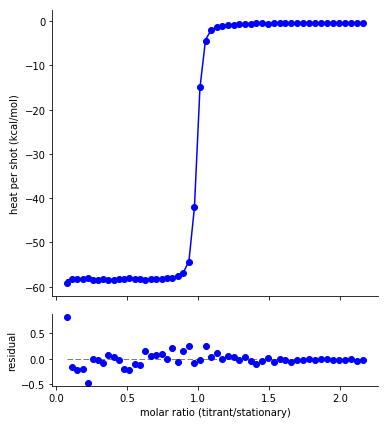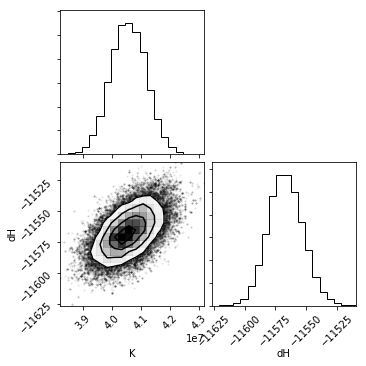# Fitting and Statistics¶

Warning

pytc will fit all sorts of complicated models to your data. It is up to you to make sure the fit is justified by the data. You should always follow best practices for selecting the your model (choosing the simplest model, inspecting residuals, knowing the assumptions made, etc.)

## Fits¶

pytc implements a variety of fitting strategies:

• BayesianFitter uses Markov-Chain Monte Carlo to estimate posterior probability distributions for all fit parameters. (Recommended)
• BootstrapFitter samples from uncertainty in each heat and then fits the model to pseudoreplicates using unweighted least-squares regression.
• MLFitter fits the model to the data using least-squares regression weighted by the uncertainty in each heat. (Default)

Details on these strategies and their implementation below.

## Fit Results¶

After you have peformed the fit, there are a variety of ways to access and assess your results. These are available for all fit strategies.

### Parameter estimates as csv¶

g.fit_as_csv


where g is a GlobalFit instance. This will print something like:

# Fit successful? True
# 2017-05-13 09:27:25.177062
# Fit type: maximum likelihood
# AIC: -96.23759423779696
# AICc: -93.8028116291013
# BIC: -84.30368995841131
# F: 292708.2585218862
# Rsq: 0.9999672039142115
# ln(L): 54.11879711889848
# num_obs: 54
# num_param: 5
# p: 1.1102230246251565e-16
type,name,exp_file,value,stdev,bot95,top95,fixed,guess,lower_bound,upper_bound
local,dilution_heat,ca-edta/tris-01.DH,1.15715e+03,4.65377e+02,2.21447e+02,2.09285e+03,False,0.00000e+00,-inf,inf
local,K,ca-edta/tris-01.DH,4.05476e+07,4.31258e+05,3.96805e+07,4.14147e+07,False,1.00000e+06,-inf,inf
local,dilution_intercept,ca-edta/tris-01.DH,-6.12671e-01,6.71064e-02,-7.47597e-01,-4.77744e-01,False,0.00000e+00,-inf,inf
local,dH,ca-edta/tris-01.DH,-1.15669e+04,1.00638e+01,-1.15872e+04,-1.15467e+04,False,-4.00000e+03,-inf,inf
local,fx_competent,ca-edta/tris-01.DH,9.73948e-01,8.86443e-05,9.73770e-01,9.74126e-01,False,1.00000e+00,-inf,inf


Lines starting with “#” are statistics and fit meta data. The statistical output is described in the statistics section below. Rendered as a table, the comma- separated output has the following form:

type name exp_file value stdev bot95 top95 fixed guess lower_bound upper_bound
local dilution_heat ca-edta/tris-01.DH 1.15715e+03 4.65377e+02 2.21447e+02 2.09285e+03 False 0.00000e+00 -inf inf
local K ca-edta/tris-01.DH 4.05476e+07 4.31258e+05 3.96805e+07 4.14147e+07 False 1.00000e+06 -inf inf
local dilution_intercept ca-edta/tris-01.DH -6.12671e-01 6.71064e-02 -7.47597e-01 -4.77744e-01 False 0.00000e+00 -inf inf
local dH ca-edta/tris-01.DH -1.15669e+04 1.00638e+01 -1.15872e+04 -1.15467e+04 False -4.00000e+03 -inf inf
local fx_competent ca-edta/tris-01.DH 9.73948e-01 8.86443e-05 9.73770e-01 9.74126e-01 False 1.00000e+00 -inf inf
• type: “local” or “global”
• name: parameter name
• exp_file: name of experiment file
• value: estimate of the parameter
• stdev: standard deviation of parameter estimate
• bot95/top95: 95% confidence intervals of parameter estimate
• fixed: “True” or “False” depending on whether parameter was held to fixed value
• guess: parameter guess
• lower_bound/upper_bound: bounds set on the fit parameter during the fit

### Fit and residuals plot¶

g.plot()


where g is a GlobalFit instance. This will create something like:The fit residuals should be randomly distributed around zero, without systematic deviations above or below. Non-random residuals can indicate that the model does not adequately describe the data, despite potentially having a small residual standard error.

### Corner plots¶

One powerful way to assess the fit results is through a corner plot, which shows the confidence on each fit parameter, as well as covariation between each parameter. The quality of the histograms is also an indication of whether you have adequate sampling when using the bootstrap or bayesian methods.

g.corner_plot()


where g is a GlobalFit instance. This will create something like:The diagonal shows a histogram for that parameter. The bottom-left cells show a 2D histogram of covariation between those parameters.

g.plot_corner uses keywords to find and filter out nuisance parameters like fx_competent or dilution_heat. To see these (or modify the filtering) change the filter_params list passed to the function.

### Statistics¶

g.fit_stats


where g is a GlobalFit instance. This will return a dictionary of fit statistics with the following keys.

• AIC: Akaike Information Criterion
• AICc: Akaike Information Criterion corrected for finite sample size
• BIC: Bayesian Information Criterion
• df: degrees of freedom
• F: The F test statistic
• ln(L): log likelihood of the model
• num_obs: number of data points
• num_param: number of floating parameters fit
• Rsq: $$R^{2}$$
• Rsq_adjusted: $$R^{2}_{adjusted}$$
• Fit type: the type of fit (maxium likelihood, bootstrap, or bayesian)
• Keys like ” bayesian: num_steps” provide information specific to a given fit type.

### Model comparison¶

Models with more parameters will generally fit the data better than models with fewer parameters. These extra parameters may or may not be meaningful. (You could, for example, fit $$N$$ data points with $$N$$ parameters. This would give a perfect fit – and very little insight into the system). A standard approach in model fittng is to choose the simplest model consistent with the data. A variety of statistics can be used to balance fitting the data well against the addition of many parameters. pytc returns four test statistics that penalize models based on the number of free parameters: Akaike Information, corrected Akaike Information, Bayesian Information, and the F-statistic.

The pytc.util.compare_models function will conveniently compare a collection of models, weighting them by AIC, AICc, and BIC.

## Fitting Strategies¶

pytc implements multiple fitting strategies including least-squares regression, bootstrap sampling + least squares, and Bayesian MCMC. These are implemented as subclasses of the pytc.fitters.Fitter. base class.

### Bayesian¶

Uses Markov-Chain Monte Carlo (MCMC) to sample from the posterior probability distributions of fit parameters. pytc uses the package emcee to do the sampling. The log likelihood function is:

$ln(L) = -0.5 \sum_{i=0}^{i < N} \Big [ \frac{(dQ_{obs,i} - dQ_{calc,i}(\vec{\theta}))^{2}}{\sigma_{i}^{2}} + ln(\sigma_{i}^{2}) \Big ]$

where $$dQ_{obs,i}$$ is an observed heat for a shot, $$dQ_{calc,i}$$ is the heat calculated for that shot by the model, and $$\sigma_{i}$$ is the experimental uncertainty on that heat.

The prior distribution is uniform within the specified parameter bounds. If any parameter is outside of its bounds, the prior is $$-\infty$$. Otherwise, the prior is 0.0 (uniform).

The posterior probability is given by the sum of the log prior and log likelihood functions.

$ln(P) = ln(L) + ln(prior)$

#### Parameter estimates¶

Parameter estimates are the means of posterior probability distributions.

#### Parameter uncertainty¶

Parameter uncertainties are estimated by numerically integrating the posterior probability distributions.

### Bootstrap¶

Samples from experimental uncertainty in each heat and then peforms unweighted least-squares regression on each pseudoreplicate using scipy.optimize.least_squares. The residuals function is:

$\vec{r} = \vec{dQ}_{obs} - \vec{dQ}_{calc}(\vec{\theta})$

where $$\vec{dQ}_{obs}$$ is a vector of the observed heats and $$\vec{dQ}_{calc}(\vec{\theta})$$ is a vector of heats observed with fit paramters $$\vec{\theta}$$.

This uses the robust Trust Region Reflective method for the nonlinear regression.

#### Parameter estimates¶

Parameter estimates are the means of bootstrap pseudoreplicate distributions.

#### Parameter uncertainty¶

Parameter uncertainties are estimated by numerically integrating the bootstrap pseudoreplicate distributions.

### Least-squares regression¶

Weighted least-squares regression using scipy.optimize.least_squares. The residuals function is:

$\vec{r} = \frac{\vec{dQ}_{obs} - \vec{dQ}_{calc}(\vec{\theta})}{\vec{\sigma}_{obs}}$

where $$\vec{dQ}_{obs}$$ is a vector of the observed heats, $$\vec{dQ}_{calc}(\vec{\theta})$$ is a vector of heats observed with fit paramters $$\vec{\theta}$$, and $$\vec{\sigma}_{obs}$$ are the uncertainties on each fit.

This uses the robust Trust Region Reflective method for the nonlinear regression.

#### Parameter estimates¶

The parameter estimates are the maximum-likelihood parameters returned by scipy.optimize.least_squares.

#### Parameter uncertainty¶

We first approximate the covariance matrix $$\Sigma$$ from the Jacobian matrix $$J$$ estimated by scipy.optimize.least_squares:

$\Sigma \approx [2(J^{T} \cdot J)]^{-1}$

We can then determine the standard deviation on the parameter estimates $$\sigma$$ by taking the square-root of the diagonal of $$\Sigma$$:

$\sigma = \sqrt(diag(\Sigma))$

Ninety-five percent confidence intervals are estimated using the Z-score assuming a normal parameter distribution with the mean and standard deviations determined above.

Warning

Going from $$J$$ to $$\Sigma$$ is an approximation. This is susceptible to numerical problems and may not always be reliable. Use common sense on your fit errors or, better yet, do Bayesian integration!